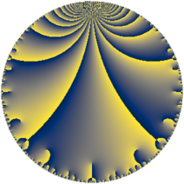# Properties

 Label 38.5.bLevel $38$ Weight $5$ Character orbit 38.b Rep. character $\chi_{38}(37,\cdot)$ Character field $\Q$ Dimension $8$ Newform subspaces $1$ Sturm bound $25$ Trace bound $0$

# Related objects

## Defining parameters

 Level: $$N$$ $$=$$ $$38 = 2 \cdot 19$$ Weight: $$k$$ $$=$$ $$5$$ Character orbit: $$[\chi]$$ $$=$$ 38.b (of order $$2$$ and degree $$1$$) Character conductor: $$\operatorname{cond}(\chi)$$ $$=$$ $$19$$ Character field: $$\Q$$ Newform subspaces: $$1$$ Sturm bound: $$25$$ Trace bound: $$0$$

## Dimensions

The following table gives the dimensions of various subspaces of $$M_{5}(38, [\chi])$$.

Total New Old
Modular forms 22 8 14
Cusp forms 18 8 10
Eisenstein series 4 0 4

## Trace form

 $$8q - 64q^{4} + 18q^{5} - 32q^{6} - 162q^{7} - 268q^{9} + O(q^{10})$$ $$8q - 64q^{4} + 18q^{5} - 32q^{6} - 162q^{7} - 268q^{9} - 6q^{11} + 512q^{16} + 510q^{17} - 12q^{19} - 144q^{20} - 396q^{23} + 256q^{24} + 3458q^{25} - 192q^{26} + 1296q^{28} - 2752q^{30} + 1002q^{35} + 2144q^{36} - 3216q^{38} - 6588q^{39} + 1376q^{42} - 8654q^{43} + 48q^{44} - 10334q^{45} + 3210q^{47} + 9222q^{49} + 9088q^{54} + 17146q^{55} - 14076q^{57} - 960q^{58} + 1314q^{61} - 15168q^{62} + 29938q^{63} - 4096q^{64} + 4928q^{66} - 4080q^{68} + 23398q^{73} + 13152q^{74} + 96q^{76} - 44622q^{77} + 1152q^{80} - 20368q^{81} + 16512q^{82} - 10440q^{83} + 21274q^{85} - 14316q^{87} + 3168q^{92} + 19416q^{93} - 34686q^{95} - 2048q^{96} - 56798q^{99} + O(q^{100})$$

## Decomposition of $$S_{5}^{\mathrm{new}}(38, [\chi])$$ into newform subspaces

Label Dim. $$A$$ Field CM Traces $q$-expansion
$$a_2$$ $$a_3$$ $$a_5$$ $$a_7$$
38.5.b.a $$8$$ $$3.928$$ $$\mathbb{Q}[x]/(x^{8} + \cdots)$$ None $$0$$ $$0$$ $$18$$ $$-162$$ $$q+\beta _{2}q^{2}+(\beta _{1}+\beta _{2})q^{3}-8q^{4}+(2-\beta _{6}+\cdots)q^{5}+\cdots$$

## Decomposition of $$S_{5}^{\mathrm{old}}(38, [\chi])$$ into lower level spaces

$$S_{5}^{\mathrm{old}}(38, [\chi]) \cong$$ $$S_{5}^{\mathrm{new}}(19, [\chi])$$$$^{\oplus 2}$$